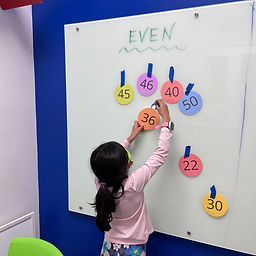Ms. Caitlyn

Target 1​

Lesson Type:

New

Number Operation

:

Number Classes

Use grouping strategies to determine if a given quantity is even or odd.

1:

Classify numbers as even or odd.

2:

Understand that even numbers can be divided into two equal groups and odd numbers cannot be divided equally into two groups.

3:

Recognize that even numbers have a 0, 2, 4, 6, or 8 in the ones’ place.

4:

Recognize that odd numbers have a 1, 3, 5, 7, or 9 in the ones’ place.

1st

Vocabulary:

Even: Equal groups of two can be made.
Odd: Equal groups of two cannot be made.

Activities:

• Students made groups of two with given numbers to determine if they were even or odd.
• Students colored in a hundreds chart to identify patterns between even and odd numbers.Home Exploration

Guiding Questions:Absent Students:

Archy

Target 2

:

1:

Use the given information in a problem to identify the correct answer.

2:

Think outside of the box to solve problems.

3:

Follow given rules to solve problems.

Vocabulary:

Activities:

• Students completed 3 x 3, 4 x 4, and 5 x 5 grids of color block sudoku.
• Students completed 3, 4, 5, 6, and 7 rings of a Tower of Hanoi puzzle.Home Exploration

Guiding Questions:Target 3

:

Vocabulary:

Activities:Home Exploration